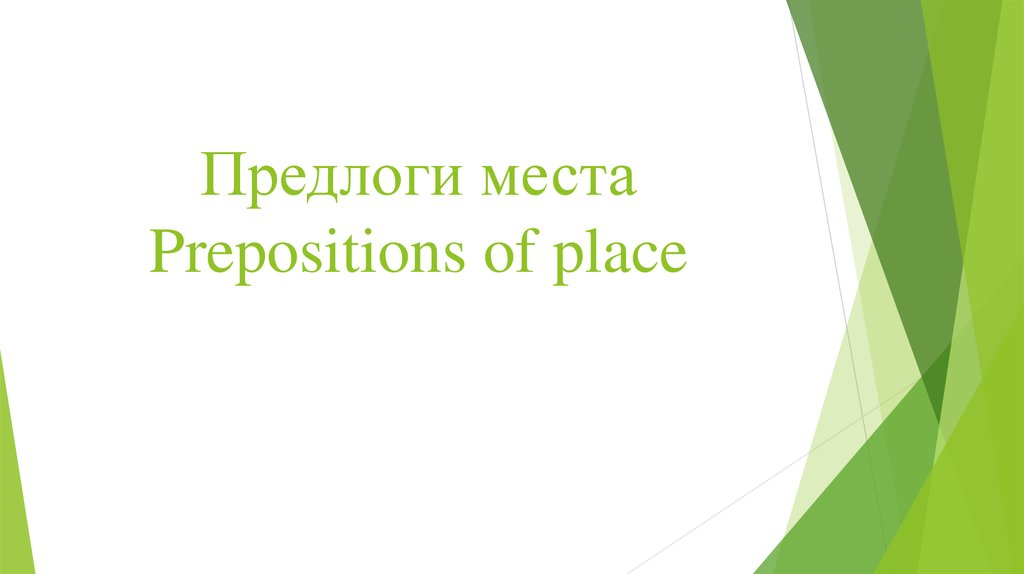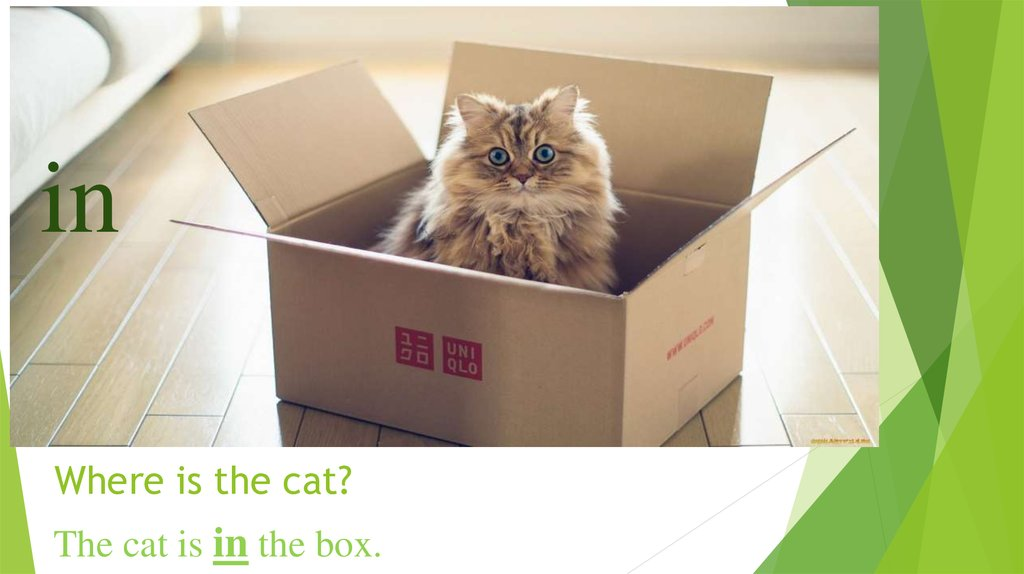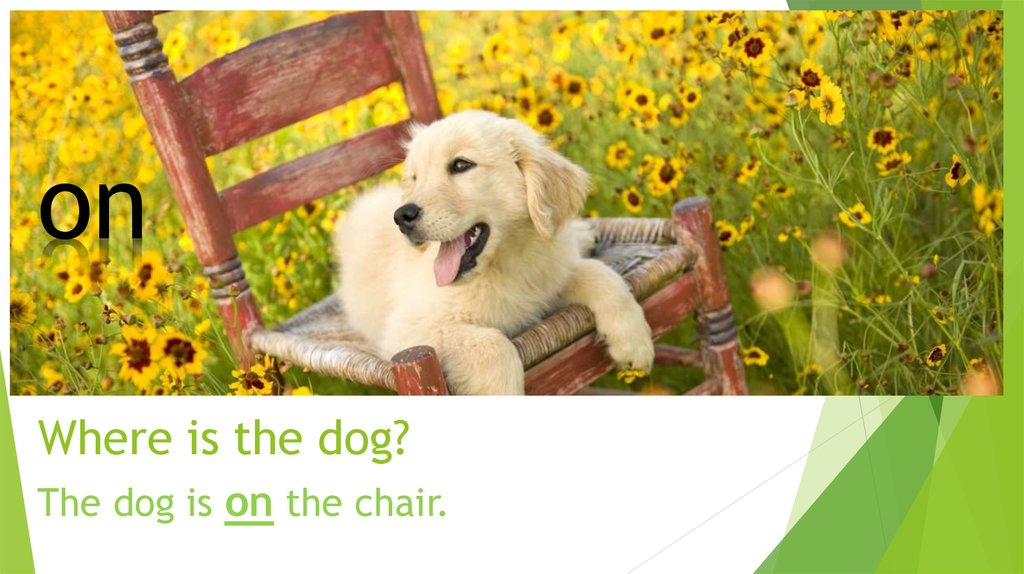Prepositions of place

2. Where is the cat?

in
Where is the cat?
The cat is in the box.

3. Where is the dog?

on
Where is the dog?
The dog is on the chair.

4. Where is the bear?

The bear is under the water.

5. Where is the rabbit?

The rabbit is behind
the box.

6. Where is the cat?

The cat is next to the fox.
Where is the fox?
The fox is next to the
cat.

7. Where is the dog?

The dog is in front of the camera.